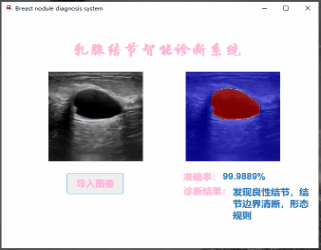# 前言

Python基本占据了深度学习领域，但是如果是新手玩家由于某个项目需要或者是想快速入门又不会Python，对训练过程及各种超参数不了解，就不妨先试试万能的Matlab，如果对Matlab语法熟悉，使用可视化工具、修改和分析学习架构，学习完Matlab的深度学习编程框架后能很快出成果，后续再深入学习，应该是一个比较好的学习过程。

Matlab学习资源直接去Mathwork：

# 一、基本介绍

1.训练集的制备
2.DeepLabV3+分割网络的构建、训练、评价
3.深度学习工具箱的使用与分类网络的构建
4.使用App Designer设计App

# 二、DeepLabV3+分割网络

## 1.语义分割网络数据集制备``````for n=1:623 %数据集样本数
Img={I};
A=Img(1);
RGB=A{1,1};
B=RGB(:,:,1);
S=size(B);
for i=1:S(1)
for j=1:S(2)
if RGB(i,j,1)==0&&RGB(i,j,2)==0&&RGB(i,j,3)==0 %三通道均为0的是其他
B(i,j)=0;
else
B(i,j)=1; %否则是结节
end
end
end
imwrite(B,[num2str(n),'.png'])
end
``````## 2.加载数据集图像和标签

``````clc,clear;
imageFolderTrain = fullfile(dataFolder,'train_image');%训练图像路径
labelFolderTrain = fullfile(dataFolder,'train_label');%训练标签路径
imageFolderVal = fullfile(dataFolder,'val_image');%验证图像路径
labelFolderVal = fullfile(dataFolder,'val_label');%验证标签路径
imdsTrain = imageDatastore(imageFolderTrain);% 加载训练图像
imdsVal = imageDatastore(imageFolderVal);% 加载验证图像
``````

``````classNames = ["Back" "Tubercle"];%设置类别名称
labelIDs = [0 1];%设置类别相应的编号
pxdsTrain = pixelLabelDatastore(labelFolderTrain,classNames,labelIDs);%生成相应的类别标签
pxdsVal = pixelLabelDatastore(labelFolderVal,classNames,labelIDs);%生成相应的类别标签
``````

## 3.DeepLabV3+网络搭建

DeepLabV3+是一种专为语义图像分割而设计的卷积神经网络 （CNN）。其他类型的语义分割网络包括全卷积网络（FCN）、SegNet、U-Net等，训练过程是一样的。

### 3.1用类加权平衡类

``````tbl = countEachLabel(pxdsTrain);%countEachLabel计算像素标签计数
frequency = tbl.PixelCount/sum(tbl.PixelCount);
bar(1:numel(classNames),frequency)
xticks(1:numel(classNames))
xticklabels(tbl.Name)
xtickangle(45)
ylabel('Frequency') %Frequency向量元素包含结节和其他的像素占比
``````
``````% 设置类别权重
imageFreq = tbl.PixelCount ./ tbl.ImagePixelCount;
classWeights = median(imageFreq) ./ imageFreq;
% 修改输出层，使用像素分类图层(pixelClassificationLayer)指定类权重
pxLayer = pixelClassificationLayer('Name','labels','Classes',tbl.Name,'ClassWeights',classWeights);
lgraph = replaceLayer(lgraph,"classification",pxLayer);
``````### 3.2创建网络

Deeplabv3+网络创建基于MobileNet v2 ，实际上是一个迁移学习的过程，最佳网络需要实证分析，这是超参数调优的另一个级别。除了MobileNet v2，还可以 ResNet-18 等，也可以尝试其他语义分割网络体系结构，如 SegNet、FCN 或 U-Net。

``````numClasses = numel(classNames);
lgraph = deeplabv3plusLayers(imageSize,numClasses,'mobilenetv2');
``````

### 3.3数据增强

``````augmenter = imageDataAugmenter('RandXTranslation',[-10 10],'RandYTranslation',[-10 10]);%翻转
pximds = pixelLabelImageDatastore(imdsTrain,pxdsTrain, ...'DataAugmentation',augmenter);
``````

### 3.4设置超参数

``````%% 设置训练参数
'LearnRateSchedule','piecewise',...
'LearnRateDropPeriod',20,...%学习率每20代降低一次
'LearnRateDropFactor',0.4,...%学习率每20代降低0.4倍
'InitialLearnRate',1e-4, ...%初始学习率
'L2Regularization',0.005, ...
'MaxEpochs',50, ...  %最大迭代次数
'MiniBatchSize',8, ...%小批量尺寸，可根据显存更改
'Shuffle','every-epoch', ...
'VerboseFrequency',2,...
'Plots','training-progress');%绘制训练曲线
``````

### 3.5开始训练并保存

`````` [net,info] = trainNetwork(pximds,lgraph,options);
save('trainedNet.mat','net');
save('trainedInfo.mat','info');
``````### 3.6网络评价

``````pxdsResults = semanticseg(imdsVal,net, ...
'MiniBatchSize',4, ...
'WriteLocation',tempdir, ...
'Verbose',false);
metrics = evaluateSemanticSegmentation(pxdsResults,pxdsVal,'Verbose',false);
metrics.DataSetMetrics %返回整个数据集、单个类和每个测试映像的各种指标
metrics.ClassMetrics %每个类的指标
````````````expectedResult = readimage(pxdsVal,10);
actual = uint8(C);
expected = uint8(expectedResult);
imshowpair(actual, expected)
``````### 3.7测试训练结果

``````%  加载训练好的模型
clc,clear;
filepath = fullfile(path,file);
imshow(I);
%Resize the test image size
I = imresize(I,[224,224]);
Segment the image.
C= semanticseg(I,net);
Display the results.

%展示分割结果
classNames = ["Back" "tubercle"];           %按顺序给出类别
cmap = camvidColorMap;                      %这里需要更改函数内参数，即指定分类区域颜色
B = labeloverlay(I,C,'ColorMap',cmap);
imshow(B),title("语义分割结果");
pixelLabelColorbar(cmap,classNames);
``````

``````function cmap = camvidColorMap()
cmap = [
0 0 255     % Back蓝
255 0 0     % Tubercle红
];
% Normalize between [0 1].
cmap = cmap ./ 255;
end
``````# 三、分类网络的建设

## 1.图像预处理

Matlab大部分搭建好的预训练网络都是三通道并且统一大小，如果不会调整参数，最简单粗暴的方法就是对训练集图像进行预处理，这里提供用Python实现批量Resize和通道转化代码供参考：

``````from PIL import Image
import os.path
import glob
def convertjpg(jpgfile, outdir, width=227, height=227):
img = Image.open(jpgfile)
try:
new_img = img.resize((width, height), Image.BILINEAR)
if new_img.mode == 'P':
new_img = new_img.convert("RGB")
if new_img.mode == 'RGBA':
new_img = new_img.convert("RGB")
new_img.save(os.path.join(outdir, os.path.basename(jpgfile)))
except Exception as e:
print(e)
print(jpgfile)

``````

``````import PIL.Image as Image
import os

save_path = r"G:\+1\5"
for i in os.listdir(path):
img = Image.open(os.path.join(path, i)).convert('RGB')
img.save(os.path.join(save_path, i))
``````

## 2.网络搭建## 3.测试训练结果

``````clc;clear
% 加载训练好的模型
% 查看模型细节
%trainedNetwork_1.Layers
% 调整图像大小
[file,path] = uigetfile('*');
image = fullfile(path,file);
file
tic%计时
% 使用模型分类
label = classify(trainedNetwork_1, I)
imshow(I);
toc
``````# 四、APP设计``````function [label,text,B] =Dec_Seg(I)
%调用模型返回三个参量
I = imresize(I,[227,227]);
[label,score] = classify(trainedNetwork_1, I);
%准确率
maxscore = max(score);
text= string(maxscore*100)+"%";
if  label == 'benign'
label='发现良性结节，结节边界清晰，形态规则';
elseif label == 'malignant'
label='发现恶性结节，结节边界欠清晰，形态欠规则';
else
label='尚未发现结节,请进一步检查' ;
end
C= semanticseg(I,net);
cmap = camvidColorMap;
B = labeloverlay(I,C,'ColorMap',cmap);
end
``````(0)